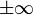# Finite facts for kids

Kids Encyclopedia Facts

If something is finite, it means it will end. The opposite is infinite. There are many uses of this word, for example:

• A finite number is a number which is not equal to$\pm\infty$; all real numbers are finite.
• A finite set is a set that has a certain number of elements.
• A finite verb is a term from linguistics that means that a verb is of a form that tells person and tense.

fr:Fini it:FinitoFinite Facts for Kids. Kiddle Encyclopedia.